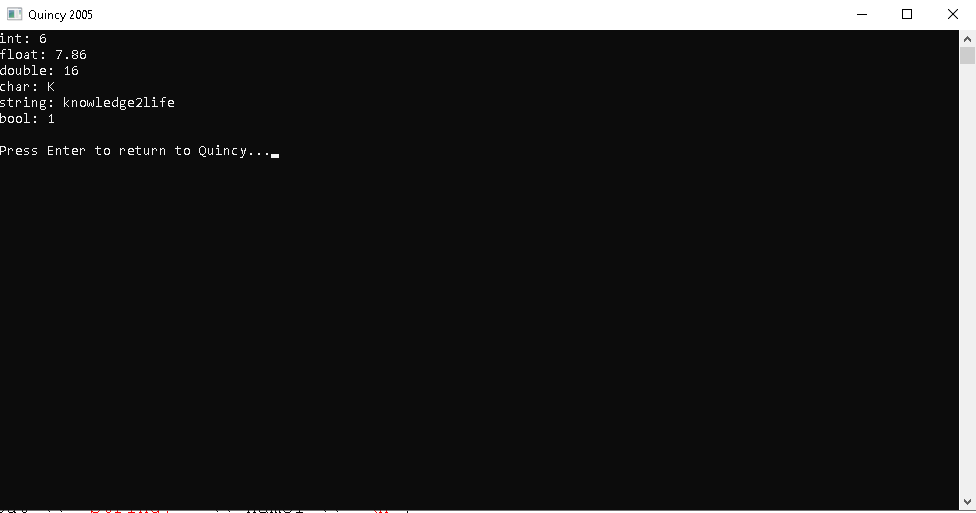# Variable data types in c++

#### Variable data types in c++

Sr.No Type & Description
1 bool
It stores true / false values
2 char
Has one byte of data value
3 int
The machine’s natural integer size
4 Float
Floating point value of single precision
5 double
Floating point value of double precision
6 void
Representation of the absence of type
7 wchar_t
A wide type of character

C++ also enables the definition of several variable kinds, including listing, display, array, reference, data structure and classes, which can be covered in following chapters.

The next section will discuss how various sorts of variables can be defined, declared and used.

#### Example

// Integer (without decimals) int x = 6; // Floating point number (with decimals storing 7 decimal digits) float y = 7.86; // Floating point number (with decimals storing 15 decimal digits) double y1 = 15.999999; // Character char ch = 'K'; // String (text) string name1 = "knowledge2life.com"; // Boolean (Stores true or false values) bool Z = true;

#### Example

#include <iostream> #include <string> using namespace std; int main () { // Creating variables // Integer (without decimals) int x = 6; // Floating point number (with decimals storing 7 decimal digits) float y = 7.86; // Floating point number (with decimals storing 15 decimal digits) double y1 = 15.999999; // Character char ch = 'K'; // String (text) string name1 = "knowledge2life"; // Boolean (Stores true or false values) bool b = true; // Print variable values cout << "int: " << x << "\n"; cout << "float: " << y << "\n"; cout << "double: " << y1 << "\n"; cout << "char: " << ch << "\n"; cout << "string: " << name1 << "\n"; cout << "bool: " <<b << "\n"; return 0; }

#### Output: